## LetsPlayMaths.Com

WELCOME TO THE WORLD OF MATHEMATICS

# Class 3 Mass

Mass

Conversion of Kilograms into Grams

Conversion of Grams into Kilograms

Subtraction of Kilograms and Grams

Multiplication of Kilograms and Grams

Division of Kilograms and Grams

Mass Test

Mass Worksheet

## Mass

We measure the mass of objects in kilograms and grams. For large quantities kilograms is used. In our day to day life we buy fruits, vegetables, meat etc. in kilograms. Small quantities or precious metals are weighed in grams. For example, Gold, silver, platinum etc. are weighed in grams.

1 kilogram = 1000 grams

The short form of kilograms is ëkgí, and the short form of gram is ëgí.

## Conversion of Kilograms into Grams

As we know 1 kilogram is equal to 1000 grams, and if we want to convert kilograms into grams then we have to multiply number of kilograms by 1000. For conversion of kilograms and grams into grams, we have to multiply the number of kilograms by 1000 and add the grams.

Example 1. Convert 5 kg into grams.

Solution. 5 kg = 5 X 1000 = 5000 g
So, 5 kg is equal to 5000 grams

Example 2. Convert 9 kg 750 g into grams

Solution. 9 kg 750 g = 9 X 1000 g + 750 g = 9000 g + 750 g = 9750 g
So, 9 kilograms 750 grams is equal to 9750 grams.

## Conversion of Grams into Kilograms

When grams are converted into kilograms and grams, the number formed by first three digits from right gives the number of
grams and the number formed by remaining digits give the number of kilograms.

Example 1. Convert 6000 grams into kilograms.

Solution. 6 0 0 0 g = 6 kg 000 g = 6 kg
So, 6000 grams is equal to 6 kg.

Example 2. Convert 22629 grams into kilograms.

Solution. 2 2 6 2 9 = 22 kg 629 g
So, 22629 grams is equal to 22 kg 629 grams

## Addition of Kilograms and Grams

This kind of addition can be done in two methods, they are given below with examples.

Method 1.

Step 1. Convert the grams into three-digit format, for example, 5 kg 8 gm should be written as 5 kg 008 gm.

Step 2. Add grams first. If the result is of 3 digits, then it should be written as grams. If the result is of 4 digits,

then the left most 1 digit should be carry over to kilogram column.

Example 1. Add 123 kg 57 g and 45 kg 245 g

Solution. Write the 123 kg 57 g and 45 kg 245 g in column format as shown below.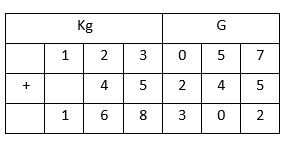Step 1. 123 kg 57 g is written as 123 kg 057 g in the table.

Step 2. Add the grams, 245 g + 057 g = 302g

Write 302 g under grams column

Step 3. Add kilograms, 123 kg + 45 Kg = 168 kg. Write 168 kg under kilogram column.

So, the answer is 168 kg 302 g.

Method 2.
We can add kilograms and grams like normal numbers, but grams should be written as three digits numbers. For example, 45 grams should be written as 045 grams and 5 grams should be written as 005 grams.

Example 1. Add 452 kg 125 g, 126 kg 457 g and 8 kg 5 g.

Solution. Write 452 kg 125 g, 126 kg 457 g and 8 kg 5 g in tabular format.So, the answer is 586 kg 587 g

## Subtraction of Kilograms and Grams

This kind of subtraction can be done in two methods, they are given below with examples.

Method 1.

Step 1. Subtract the grams first. If we had to borrow 1 from kilogram, then it must be converted to grams first that is 1000 g

and then perform the subtraction.

Step 2. Subtract the kilograms. If we borrowed 1 kg for grams subtraction, then we must reduce 1 kg from the kilogram.

Example 1. Subtract 78 kg 750 g from 197 kg 250 g

Solution. Arrange 197kg 250 g and 78 kg 750 g in tabular format.Step 1. Subtract grams. 750 g > 250 g, so we have to borrow 1 kg from 197 kg.

1 kg + 250 g = 1000 g + 250 g = 1250 g

1250 g ñ 750 g = 500 g, Write 500 g under grams column.

Step 2. Since we borrowed 1 kg, so 197 kg becomes 196 kg. Now subtract 78 kg from 196 kg.

196 kg ñ 78 kg = 118 kg. Write 118 kg under kilograms column.

So, the answer is 118 kg 500 g.

Method 2.
We can subtract kilograms and grams as normal subtraction. But, grams should be written as three-digit numbers.

Example 1. Subtract 426 kg 546 g from 526 kg 126 g.

Solution. Write 426 kg 546 g and 526 kg 126 g in tabular format.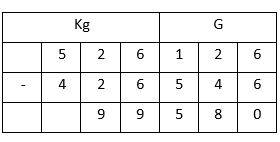So, the answer is 99 kg 580 g

## Multiplication of Kilograms and Grams

Multiplication of kilograms and grams can be done in two methods.

Method 1.
Step 1. Multiply grams first. If the result is consisting of three digits then write it under grams column. But, if the result is of four digits then carry the 1st digit from left to kilogram columns for addition.

Step 2. Multiply kilograms, add the result with carryover from the grams column.

Example 1. Multiply 82 kg 135 g by 6.

Solution. Write the numbers in tabular format as show below.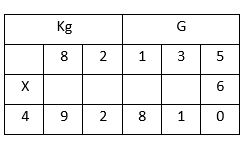Step 1. Multiply grams first. 135 g X 6 = 810 g

Write 810 g under grams column.

Step 2. Multiply kilograms next. 82 kg X 6 = 492 kg
Write 492 kg under kilogram column.
So, the answer is 492 kg 810 g.

Method 2.
We can multiply kilograms and grams as normal multiplication. But, grams should be written as three-digit numbers.

Example 1. Multiply 326 kg 9 g by 4

Solution. Write 326 kg 9 g and 4 in tabular format as shown below.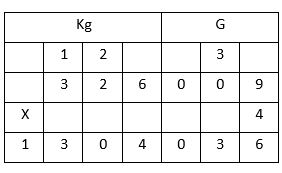So, the answer is 1304 kg 36 g.

## Division of Kilograms and Grams

Division of kilograms and grams can be done in two methods.

Method 1.

Step 1. Convert kilograms and grams into grams.

Step 2. Divide the grams with divisor like normal division.

Step 3. Now convert the quotient to kilograms and grams.

Example 1. Divide 94kg 464 by 4

Solution. 94 kg 464 g is equals to 94464 gSo, the answer is 23 kg 616 g.

Method 2.
Division of kilograms and grams can be done with normal division. In this method, grams must be written in 3-digit format.

Example 1. Divide 26 kg 525 g by 5

Solution.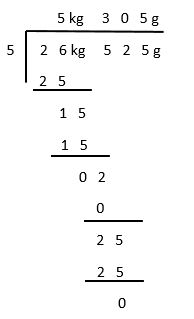So, the answer is 5 kg 305 g.

Mass Test - 1

Mass Test - 2

## Class-3 Mass Worksheet

Mass Worksheet - 1